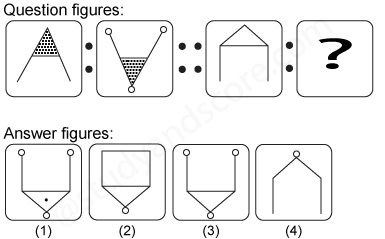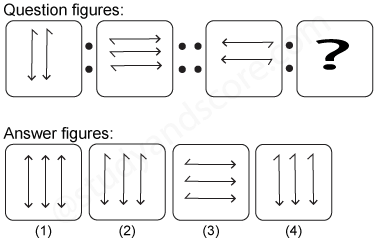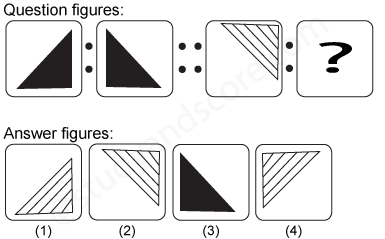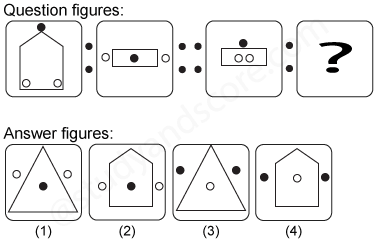# Analogy- Test 2

1)

Select the related figure from the given answer figures.

[SSC CGL, 2002]2)

Select the related figure from the given answer figures.

[SSC CGL, 2002]3)

Select the related figure from the given answer figures.

[SSC CGL, 2003]4)

Select the related figure from the given answer figures.

[SSC CGL, 2003]5)

Select the related figure from the given answer figures.

[SSC CGL, 2003]--Share with your friends --